# Apply function to every value in R dataframe

• Last Updated : 05 Apr, 2021

In R Programming Language to apply a function to every integer type value in a data frame, we can use lapply function from dplyr package. And if the datatype of values is string then we can use paste() with lapply. Let’s understand the problem with the help of an example.

Dataset in use:

after applying value*7+1 to each value of the dataframe

Expected result:

### Method 1 :  Using lapply function:

lapply is a function from apply family. By using lapply, we can avoid for loop as for loop is slower than lapply. lapply works faster than a normal loop because it doesn’t mess with the environment you work in. It returns output as a list. ‘l’ in lapply indicates list.

Syntax:

lapply(X, FUN, …)

Here, X can be a vector list or data frame. And FUN takes a function that you wish to apply to the data frame as an argument.

Approach:

• Create a dummy dataset.
• Create a custom function that you want to apply to every value in the data frame.
• Apply this custom function to every value in the data frame with the help of lapply.
• Display result

Example

## R

 `# Apply function to every value in dataframe`` ` `# Creating dataset ``m <- ``c``(1,9,6,5,9,6)`` ` `n <- ``c``(8,2,3,6,4,3)`` ` `o <- ``c``(21,0,14,5,3,2)`` ` `p <- ``c``(4,6,3,7,1,3)`` ` `# creating dataframe``df <- ``data.frame``(A=m,B=n,C=o,D=p)`` ` `# creating function``# that will multiply ``# each value by 7 and then add 1``magic_fun <- ``function``(x){``  ``return ``(x*7+1)}`` ` `# applying the custom function to every value and converting ``# it to dataframe, as lapply returns result in list ``# we have to convert it to data frame``data.frame``(``lapply``(df,magic_fun))`

Output :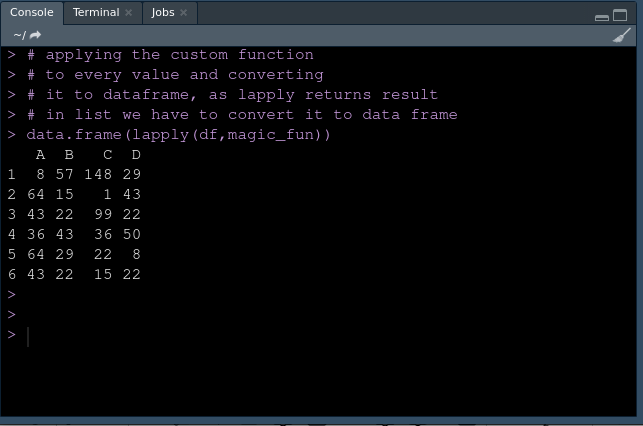Using lapply

### Method 2 : Using paste and apply function:

paste() takes an R object as an argument and converts it to characters then paste it back with another string, i.e.it converts the argument to the character string and concatenates them.

Syntax:

paste (…, sep = ” “)

Our R object which is to be converted to string goes in place of “…”,  sep=” “  represents a character string to separate the terms.

Approach:

• Create a dummy dataset.
• Apply the custom function which will print “Hello,” then value in the data frame value
• Display result

Example:

## R

 `# Apply function to every value in dataframe`` ` `# Creating dataset ``m <- ``c``(``"Vikas"``,``"Varun"``,``"Deepak"``)`` ` `n <- ``c``(``"Komal"``,``"Suneha"``,``"Priya"``)`` ` `# creating dataframe``df <- ``data.frame``(A=m,B=n)`` ` `# Applying custom function to every element in dataframe``df[]<-``data.frame``(``lapply``(df,``function``(x) ``paste``(``"Hello,"``,x,sep=``""``)))`` ` `# display dataset``df`

Output: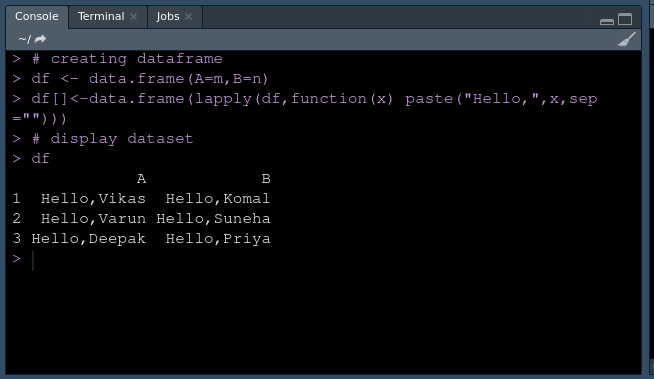Using paste and apply

### Method 3: Using purrr

purrr is a functional programming toolkit. Which comes with many useful functions such as a map. The map() function iterates across all entries of the vector and returns the output as a list. It allows us to replace for loop with in the code and makes it easier to read.

Syntax :

map(.x, .f) returns a list

map_df(.x, .f) returns a data frame

map_dbl(.x, .f) returns a numeric (double) vector

map_chr(.x, .f) returns a character vector

map_lgl(.x, .f) returns a logical vector

Here, .x is input and .f is a function that you want to be applied. Input to map function can be a list, a vector, or a data frame.

Note: You need to install purrr package explicitly using the following command.

install.packages(“purrr”)

Approach :

• Create a vector, a list and a data frame
• Create a custom function which you want to be applied.
• Use map() to apply custom function on vector, list and data frame.
• Display result

Working with integer datatype:

Program:

## R

 `# Apply function to every value in dataframe`` ` `# install and load the purrr``install.packages``(``"purrr"``)``library``(``"purrr"``)`` ` `# Creating a vector``x <- ``c``(1,2,3,4,5,7,8,9)`` ` `# creating list``y <- ``list``(2,4,9,6,3,7,1,5,4)`` ` `# creating dataframe``df <- ``data.frame``(A=``c``(1,2,3,4,5),B=``c``(6,7,8,9,10))`` ` `# creating custom function``custom_f <- ``function``(x){``  ``return``(x*2)``}`` ` `# applying function to vector``# and return output as vector``map_dbl``(x,.f=custom_f)`` ` `# applying function to list``# and return output as vector``map``(y,.f=custom_f)`` ` `# applying function to dataframe``# and return output as dataframe``map_df``(df,.f=custom_f)`

Output:

For a vector :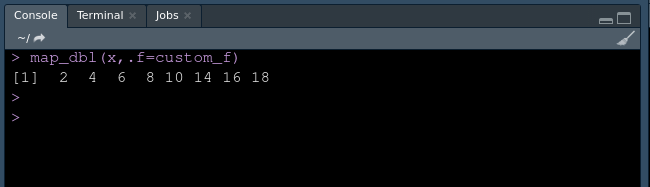Vector Output

For a list :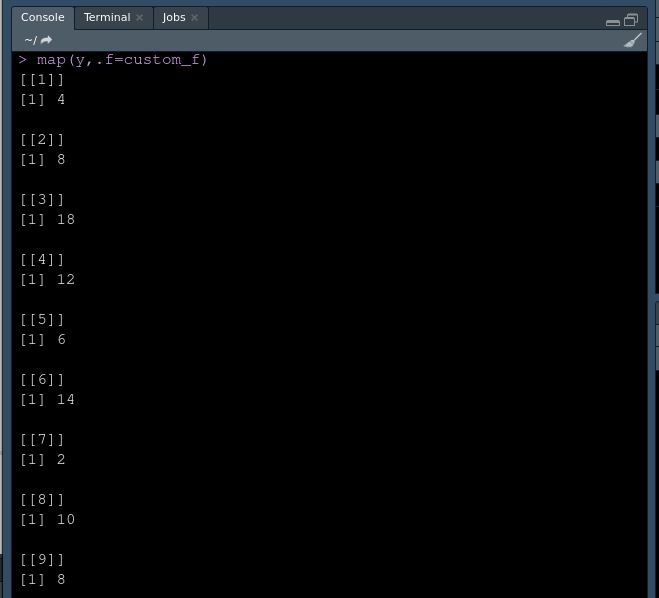List output

For a data frame: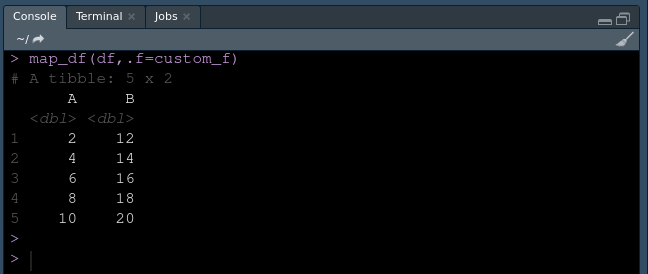dataframe output

Working with character datatype:

Program:

## R

 `# Apply function to every value in dataframe`` ` `#install and load the purrr``#install.packages("purrr")``library``(``"purrr"``)`` ` `# Creating a vector``x <- ``c``(``"Red"``,``"Blue"``, ``"Green"``,``"Yellow"``,``"Orange"``)`` ` `# creating list``y <- ``list``(``"spring"``,``"summer"``, ``"fall"``,``"winter"``)`` ` `# creating dataframe``df <- ``data.frame``(Working_days=``c``(``"Mon"``,``"Tues"``,``"Wednes"``),``                  ``off_days=``c``(``"Sun"``,``"Satur"``,``"Thurs"``) )               `` ` `# applying function to vector``# and return output as vector``map_chr``(x,paste0,``" color"``)`` ` `# applying function to list``# and return output as vector``map``(y,paste0,``" season"``)`` ` `# applying function to dataframe``# and return output as dataframe``map_df``(df,paste0,``"day"``)`

Output:

For a vector: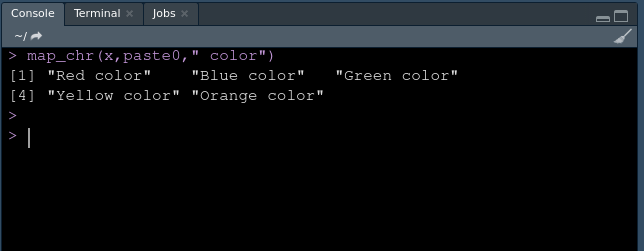Vector as Output

For a list: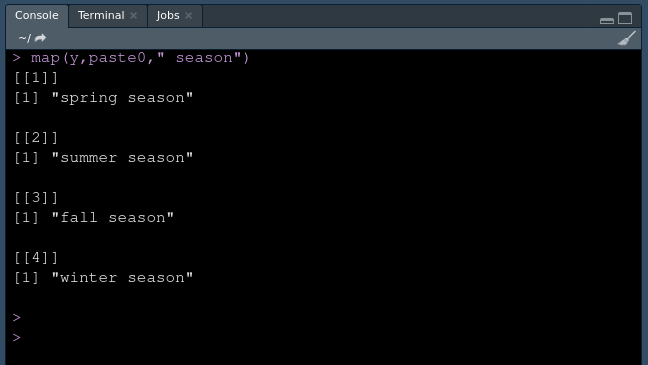List as Output

For a data frame :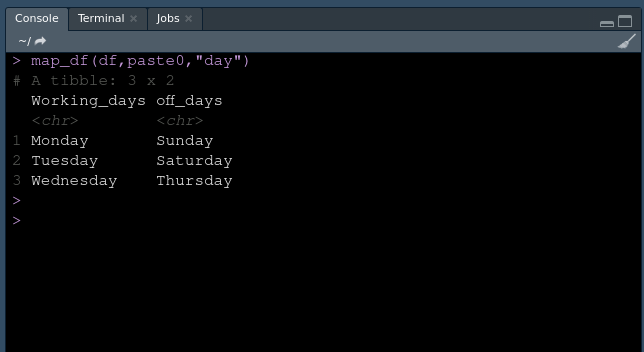Dataframe as Output

My Personal Notes arrow_drop_up